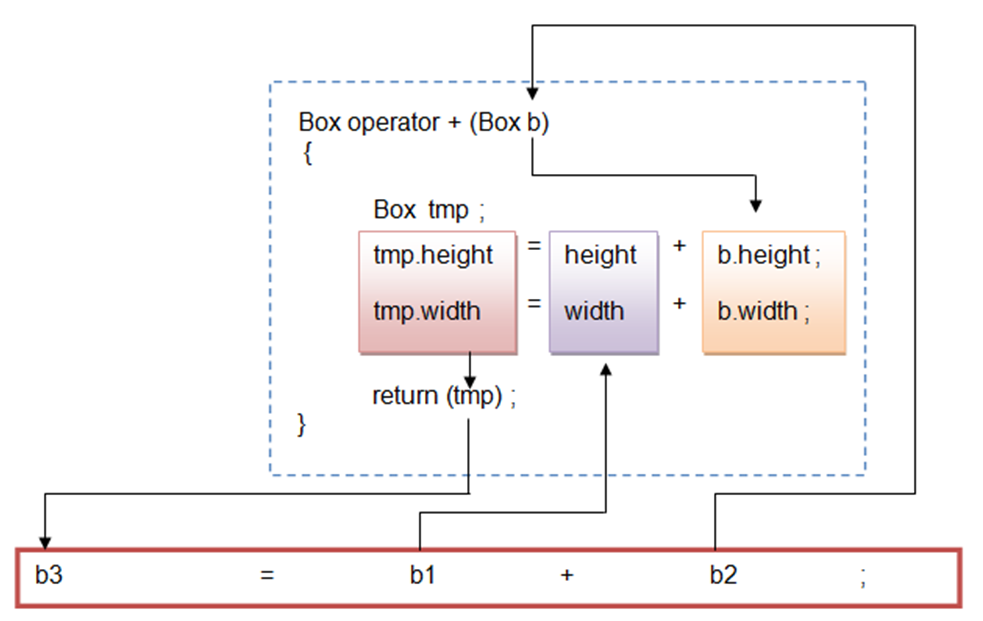• We can overload the binary operator just like the unary operators either using member function or friend function.

• If we are using member function for overloading binary operator then we have to pass only one argument to the operator function.
• Consider the example given below of class ‘Box’ in which we are overloading operator ‘+’ to add the two objects of ‘Box’ class and create a new object which has sum of height and width of both previously created objects.
``````/* Program to overload binary operator + using member function */

#include "iostream.h"
#include "conio.h"

class Box
{
double width;
double height;

public :
Box() ;  // default constructor
Box(double,double) ; // constructor with parameter

void show() ;
};

Box :: Box ()
{
width = height = 0.0 ;
}

Box :: Box (double w, double h)
{
width = w ;
height = h ;
}
// Operator function definition
Box Box :: operator +(Box b)
{
Box tmp;   // create temporary object

tmp.height = height + b.height;
tmp.width  =  width + b.width;
return(tmp);
}

void Box :: show ()
{
cout<<"Width : "<<width <<endl ;
cout<<"Height : "<<height <<endl ;
}
void main()
{
Box b1,b2,b3;

b1 = Box(10,20) ;
b2 = Box(10,20) ;

b1.show() ;  // show object 1
b2.show() ; // show object 2

// Call operator overload function + (This method is called by the object r1)
b3 = b1 + b2;  // implicit call
b3.show();    // show object 3 after addition of both objects
}``````
• In above example we have overloaded ‘+’ operator using member function.
• We have to keep in mind the following points for the above example:
1. An Operator function ‘operator +’ has one argument of type object of class ‘Box’.
2. An Operator function returns the object of type ‘Box’.
3. It is a member function of class ‘Box’.
• We have called the operator function by using the following statement:
``b3 = b1 + b2;``
• Here object ‘b1’ calls the function ‘operator +’ and object ‘b2’  is passed as argument.
• The operator function returns the object and assigned to object ‘b3’.
``b3 = b2 + b1 ;``
• In above statement, object ‘b2’ calls the function ‘operator +’ and object ‘b1’  is passed as argument.
• In short, in operator overloading,  left operator takes the responsibility to invoke the operator function and right operator is passed as an argument.
• We can also call the operator function by the following way.
``b3 = b1.opeator + (b2); //calling like normal function. Explicit call ``
• The following is a graphical representation of what happens when operator overloading function is invoked.• If we are using friend function to overload binary operator, we have to pass two arguments to operator function.
• Consider the previous example of class ‘Box’ in which we are overloading operator ‘+’ to add the two objects of ‘Box’ class and create a new object which has sum of height and width of both previously created objects.
• The same can be achieved for overloading binary operator “+” using friend function as below:
``````// Program to overload binary operator + using friend function

#include "iostream.h"
#include "conio.h"

class Box
{
double width;
double height;

public :
Box() ;  // default constructor
Box(double,double) ; // constructor with parameter

void show() ;
};

Box :: Box ()
{
width = height = 0.0 ;
}

Box :: Box (double w, double h)
{
width = w ;
height = h ;
}
// Operator function definition
Box operator +(Box b1, Box b2)
{
Box tmp;   // create temporary object

tmp.height = b1.height + b2.height;
tmp.width  =  b1.width + b2.width;
return(tmp);
}

void Box :: show ()
{
cout<<"Width : "<<width <<endl ;
cout<<"Height : "<<height <<endl ;
}
void main()
{
Box b1,b2,b3;

b1 = Box(10,20) ;
b2 = Box(10,20) ;

b1.show() ;  // show object 1
b2.show() ; // show object 2

// Call operator overload function + (This method is called by the object r1)
b3 = b1 + b2;  // implicit call
b3.show();    // show object 3 after addition of both objects
}``````
• In above example we have overloaded ‘+’ operator using friend function.
• We have to keep in mind the following points for the above example:
1. An Operator function ‘operator +’ has two arguments of type object of class ‘Box’.
2. An Operator function returns the object of type ‘Box’.
3. It is a friend function.
• We can call the operator function using the following statement:
``b3 = b1 + b2;  // implicit call using expression syntax``
• Here we are implicitly calling operator function and passing two objects of class ‘Box’ as arguments. The operator function returns the object and assigned to object ‘b3’.
• We can also call the operator function explicitly by the following way.
``b3 = operator + (b1, b2); // explicit call using normal function call``